# NCERT solutions for Class 7 Maths chapter 15 - Visualising Solid Shapes [Latest edition]

#### Chapters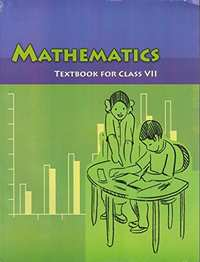## Chapter 15: Visualising Solid Shapes

Exercise 15.1Exercise 15.2Exercise 15.3Exercise 15.4
Exercise 15.1 [Pages 281 - 282]

### NCERT solutions for Class 7 Maths Chapter 15 Visualising Solid Shapes Exercise 15.1 [Pages 281 - 282]

Exercise 15.1 | Q 1.1 | Page 281

Identify the nets which can be used to make cubes (cut out copies of the nets and try it):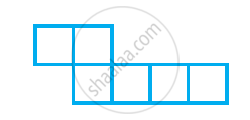Exercise 15.1 | Q 1.2 | Page 281

Identify the nets which can be used to make cubes (cut out copies of the nets and try it):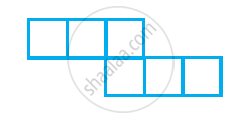Exercise 15.1 | Q 1.3 | Page 281

Identify the nets which can be used to make cubes (cut out copies of the nets and try it):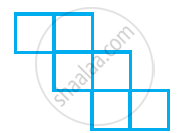Exercise 15.1 | Q 1.4 | Page 281

Identify the nets which can be used to make cubes (cut out copies of the nets and try it):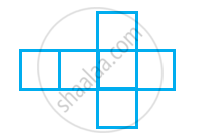Exercise 15.1 | Q 1.5 | Page 281

Identify the nets which can be used to make cubes (cut out copies of the nets and try it):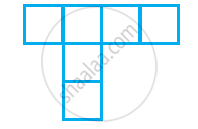Exercise 15.1 | Q 1.6 | Page 281

Identify the nets which can be used to make cubes (cut out copies of the nets and try it):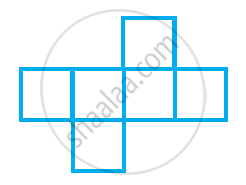Exercise 15.1 | Q 2 | Page 281

Dice are cubes with dots on each face. Opposite faces of a die always have a total of seven dots on them.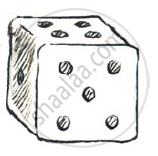Here are two nets to make dice (cubes); the numbers inserted in each square indicate
the number of dots in that box.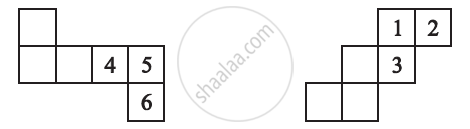Insert suitable numbers in the blanks, remembering that the number on the
opposite faces should total to 7.

Exercise 15.1 | Q 3 | Page 281

Can this be a net for a die?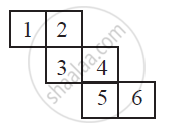Exercise 15.1 | Q 4 | Page 282

Here is an incomplete net for making a cube. Complete it in at least two different ways. Remember that a cube has six faces. How many are there in the net here? (Give two separate diagrams. If you like, you may use a squared sheet for easy manipulation.)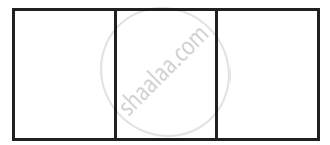Exercise 15.1 | Q 5 | Page 282

Match the nets with appropriate solids:

 a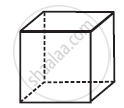1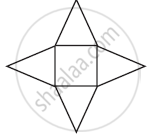b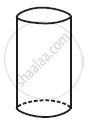2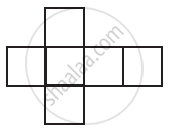c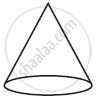3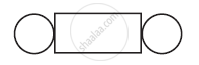d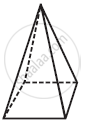4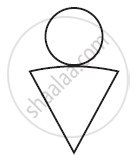Exercise 15.2 [Pages 285 - 286]

### NCERT solutions for Class 7 Maths Chapter 15 Visualising Solid Shapes Exercise 15.2 [Pages 285 - 286]

Exercise 15.2 | Q 1.1 | Page 285

Use isometric dot paper and make an isometric sketch for of the given shapes: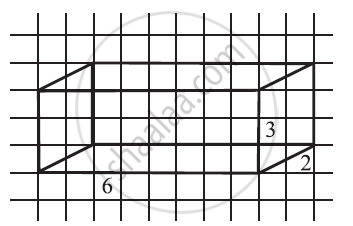Exercise 15.2 | Q 1.2 | Page 285

Use isometric dot paper and make an isometric sketch for of the given shapes: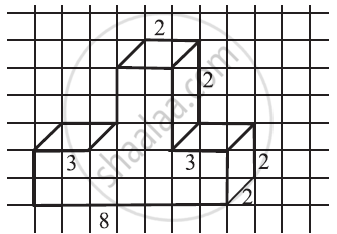Exercise 15.2 | Q 1.3 | Page 285

Use isometric dot paper and make an isometric sketch for of the given shapes: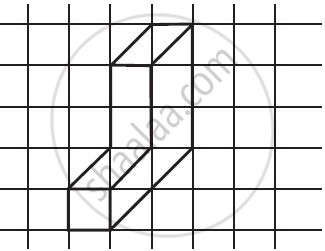Exercise 15.2 | Q 1.4 | Page 285

Use isometric dot paper and make an isometric sketch for of the given shapes: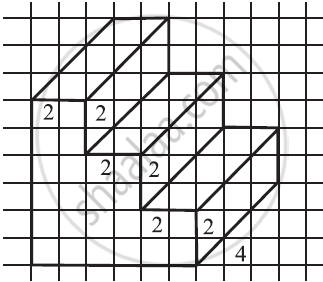Exercise 15.2 | Q 2 | Page 285

The dimensions of a cuboid are 5 cm, 3 cm and 2 cm. Draw three different isometric sketches of this cuboid

Exercise 15.2 | Q 3 | Page 285

Three cubes each with 2 cm edge are placed side by side to form a cuboid. Sketch an oblique or isometric sketch of this cuboid.

Exercise 15.2 | Q 4 | Page 285

Make an oblique sketch for each one of the given isometric shapes: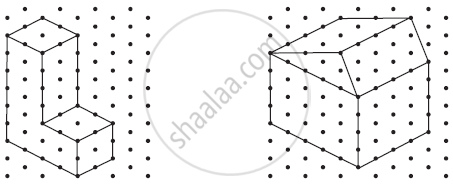Exercise 15.2 | Q 5.1 | Page 286

Give an oblique sketch of the following:

1) A cuboid of dimensions 5 cm, 3 cm and 2 cm. (Is your sketch unique?)

2) A cube with an edge 4 cm long.

Exercise 15.2 | Q 5.2 | Page 286

Give an isometric sketch for the following:

1) A cuboid of dimensions 5 cm, 3 cm and 2 cm. (Is your sketch unique?)

2) A cube with an edge 4 cm long.

Exercise 15.3 [Page 288]

### NCERT solutions for Class 7 Maths Chapter 15 Visualising Solid Shapes Exercise 15.3 [Page 288]

Exercise 15.3 | Q 1.1 | Page 288

What cross-sections do you get when you give a vertical cut and horizontal cut to the given solids a brick.

Exercise 15.3 | Q 1.2 | Page 288

What cross-sections do you get when you give a vertical cut and horizontal cut to the given solids a round apple.

Exercise 15.3 | Q 1.3 | Page 288

What cross-sections do you get when you give a vertical cut and horizontal cut to the given solids a die.

Exercise 15.3 | Q 1.4 | Page 288

What cross-sections do you get when you give a vertical cut and horizontal cut to the given solids a circular pipe.

Exercise 15.3 | Q 1.5 | Page 288

What cross-sections do you get when you give a vertical cut and horizontal cut to the given solids a ice cream cone.

Exercise 15.4 [Pages 289 - 290]

### NCERT solutions for Class 7 Maths Chapter 15 Visualising Solid Shapes Exercise 15.4 [Pages 289 - 290]

Exercise 15.4 | Q 1. (i) | Page 289

A bulb is kept burning just right above the following solid. Name the shape of the shadow obtained in case. Attempt to give a rough sketch of the shadow. (You may try to experiment first and then answer these question).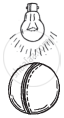A ball

Exercise 15.4 | Q 1. (ii) | Page 289

A bulb is kept burning just right above the following solid. Name the shape of the shadow obtained in case. Attempt to give a rough sketch of the shadow. (You may try to experiment first and then answer these question).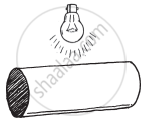A cylindrical pipe

Exercise 15.4 | Q 1. (iii) | Page 289

A bulb is kept burning just right above the following solid. Name the shape of the shadow obtained in case. Attempt to give a rough sketch of the shadow. (You may try to experiment first and then answer these question).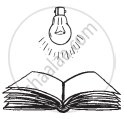A book

Exercise 15.4 | Q 2. (i) | Page 289

Here are the shadow of some 3-D object, when seen under the lamp of an overhead projector. Identify the solid(s) that match shadow. (There may be multiple answer for these!)

A circle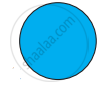Exercise 15.4 | Q 2. (ii) | Page 289

Here are the shadow of some 3-D object, when seen under the lamp of an overhead projector. Identify the solid(s) that match shadow. (There may be multiple answer for these!)

A squareExercise 15.4 | Q 2. (iii) | Page 289

Here are the shadow of some 3-D object, when seen under the lamp of an overhead projector. Identify the solid(s) that match shadow. (There may be multiple answer for these!)

A triangle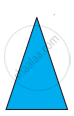Exercise 15.4 | Q 2. (iv) | Page 289

Here are the shadow of some 3-D object, when seen under the lamp of an overhead projector. Identify the solid(s) that match shadow. (There may be multiple answer for these!)

A rectangle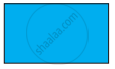Exercise 15.4 | Q 3. (i) | Page 290

Examine if the following are true statement:

The cube can cast a shadow in the shape of a rectangle.

• True

• false

Exercise 15.4 | Q 3. (ii) | Page 290

Examine if the following are true statement:

The cube can cast a shadow in the shape of a hexagon.

• true

• false

## Chapter 15: Visualising Solid Shapes

Exercise 15.1Exercise 15.2Exercise 15.3Exercise 15.4## NCERT solutions for Class 7 Maths chapter 15 - Visualising Solid Shapes

NCERT solutions for Class 7 Maths chapter 15 (Visualising Solid Shapes) include all questions with solution and detail explanation. This will clear students doubts about any question and improve application skills while preparing for board exams. The detailed, step-by-step solutions will help you understand the concepts better and clear your confusions, if any. Shaalaa.com has the CBSE Class 7 Maths solutions in a manner that help students grasp basic concepts better and faster.

Further, we at Shaalaa.com provide such solutions so that students can prepare for written exams. NCERT textbook solutions can be a core help for self-study and acts as a perfect self-help guidance for students.

Concepts covered in Class 7 Maths chapter 15 Visualising Solid Shapes are Plane Figures and Solid Shapes, Nets for Building 3-d Shapes - Cube, Cuboids, Cylinders, Cones, Pyramid, and Prism, Drawing Solids on a Flat Surface - Oblique Sketches, Drawing Solids on a Flat Surface - Isometric Sketches, Visualising Solid Objects, Viewing Different Sections of a Solid, Faces, Edges and Vertices.

Using NCERT Class 7 solutions Visualising Solid Shapes exercise by students are an easy way to prepare for the exams, as they involve solutions arranged chapter-wise also page wise. The questions involved in NCERT Solutions are important questions that can be asked in the final exam. Maximum students of CBSE Class 7 prefer NCERT Textbook Solutions to score more in exam.

Get the free view of chapter 15 Visualising Solid Shapes Class 7 extra questions for Class 7 Maths and can use Shaalaa.com to keep it handy for your exam preparation